# 11  Binary Logistic Regression Case Study 1

Case Study in Binary Logistic Regression, Model Selection and Approximation: Predicting Cause of Death

## 11.1 Overview

This chapter contains a case study on developing, describing, and validating a binary logistic regression model. In addition, the following methods are exemplified:

1. Data reduction using incomplete linear and nonlinear principal components
2. Use of AIC to choose from five modeling variations, deciding which is best for the number of parameters
3. Model simplification using stepwise variable selection and approximation of the full model
4. The relationship between the degree of approximation and the degree of predictive discrimination loss
5. Bootstrap validation that includes penalization for model uncertainty (variable selection) and that demonstrates a loss of predictive discrimination over the full model even when compensating for overfitting the full model.

The data reduction and pre-transformation methods used here were discussed in more detail in Chapter 8. Single imputation will be used because of the limited quantity of missing data.

## 11.2 Background

Consider the randomized trial of estrogen for treatment of prostate cancer described in Chapter 8. In this trial, larger doses of estrogen reduced the effect of prostate cancer but at the cost of increased risk of cardiovascular death. Kay (1986) did a formal analysis of the competing risks for cancer, cardiovascular, and other deaths. It can also be quite informative to study how treatment and baseline variables relate to the cause of death for those patients who died. We subset the original dataset of those patients dying from prostate cancer ($$n=130$$), heart or vascular disease ($$n=96$$), or cerebrovascular disease ($$n=31$$). Our goal is to predict cardiovascular–cerebrovascular death (cvd, $$n=127$$) given the patient died from either cvd or prostate cancer. Of interest is whether the time to death has an effect on the cause of death, and whether the importance of certain variables depends on the time of death.

## 11.3 Data Transformations and Single Imputation

In R, first obtain the desired subset of the data and do some preliminary calculations such as combining an infrequent category with the next category, and dichotomizing ekg for use in ordinary principal components (PCs).

Code
require(rms)
options(prType='html')
getHdata(prostate)
prostate <-
within(prostate, {
levels(ekg)[levels(ekg) %in%
c('old MI','recent MI')] <- 'MI'
ekg.norm <- 1*(ekg %in% c('normal','benign'))
levels(ekg) <- abbreviate(levels(ekg))
pfn <- as.numeric(pf)
levels(pf)  <- levels(pf)[c(1,2,3,3)]
cvd <- status %in% c("dead - heart or vascular",
rxn = as.numeric(rx) })
# Use transcan to compute optimal pre-transformations
ptrans <-   # See Figure (* @fig-prostate-transcan*)
transcan(~ sz + sg + ap + sbp + dbp +
age + wt + hg + ekg + pf + bm + hx + dtime + rx,
imputed=TRUE, transformed=TRUE,
data=prostate, pl=FALSE, pr=FALSE)
# Use transcan single imputations
imp <- impute(ptrans, data=prostate, list.out=TRUE)


Imputed missing values with the following frequencies
and stored them in variables with their original names:

sz  sg age  wt ekg
5  11   1   2   8 
Code
NAvars <- all.vars(~ sz + sg + age + wt + ekg)
for(x in NAvars) prostate[[x]] <- imp[[x]]
subset <- prostate$status %in% c("dead - heart or vascular", "dead - cerebrovascular","dead - prostatic ca") trans <- ptrans$transformed[subset,]
psub  <- prostate[subset,]

## 11.4 Regression on Original Variables, Principal Components and Pretransformations

We first examine the performance of data reduction in predicting the cause of death, similar to what we did for survival time in Section Section 8.6. The first analyses assess how well PCs (on raw and transformed variables) predict the cause of death.

There are 127 cvds. We use the 15:1 rule of thumb discussed in Section 4.4 to justify using the first 8 PCs. ap is log-transformed because of its extreme distribution.

Code
# Function to compute the first k PCs
ipc <- function(x, k=1, ...)
princomp(x, ..., cor=TRUE)$scores[,1:k] # Compute the first 8 PCs on raw variables then on # transformed ones pc8 <- ipc(~ sz + sg + log(ap) + sbp + dbp + age + wt + hg + ekg.norm + pfn + bm + hx + rxn + dtime, data=psub, k=8) f8 <- lrm(cvd ~ pc8, data=psub) pc8t <- ipc(trans, k=8) f8t <- lrm(cvd ~ pc8t, data=psub) # Fit binary logistic model on original variables f <- lrm(cvd ~ sz + sg + log(ap) + sbp + dbp + age + wt + hg + ekg + pf + bm + hx + rx + dtime, data=psub) # Expand continuous variables using splines g <- lrm(cvd ~ rcs(sz,4) + rcs(sg,4) + rcs(log(ap),4) + rcs(sbp,4) + rcs(dbp,4) + rcs(age,4) + rcs(wt,4) + rcs(hg,4) + ekg + pf + bm + hx + rx + rcs(dtime,4), data=psub) # Fit binary logistic model on individual transformed var. h <- lrm(cvd ~ trans, data=psub) The five approaches to modeling the outcome are compared using AIC (where smaller is better). Code c(f8=AIC(f8), f8t=AIC(f8t), f=AIC(f), g=AIC(g), h=AIC(h))  f8 f8t f g h 257.6573 254.5172 255.8545 263.8413 254.5317  Based on AIC, the more traditional model fitted to the raw data and assuming linearity for all the continuous predictors has only a slight chance of producing worse cross-validated predictive accuracy than other methods. The chances are also good that effect estimates from this simple model will have competitive mean squared errors. ## 11.5 Description of Fitted Model Here we describe the simple all-linear full model. Summary statistics and a Wald-ANOVA table are below, followed by partial effects plots with pointwise confidence bands, and odds ratios over default ranges of predictors. Code spar(ps=8) f Logistic Regression Model  lrm(formula = cvd ~ sz + sg + log(ap) + sbp + dbp + age + wt + hg + ekg + pf + bm + hx + rx + dtime, data = psub)  Model Likelihood Ratio Test Discrimination Indexes Rank Discrim. Indexes Obs 257 LR χ2 144.39 R2 0.573 C 0.893 FALSE 130 d.f. 21 R221,257 0.381 Dxy 0.786 TRUE 127 Pr(>χ2) <0.0001 R221,192.7 0.473 γ 0.786 max |∂log L/∂β| 5×10-11 Brier 0.133 τa 0.395 β S.E. Wald Z Pr(>|Z|) Intercept -4.5130 3.2210 -1.40 0.1612 sz -0.0640 0.0168 -3.80 0.0001 sg -0.2967 0.1149 -2.58 0.0098 ap -0.3927 0.1411 -2.78 0.0054 sbp -0.0572 0.0890 -0.64 0.5201 dbp 0.3917 0.1629 2.40 0.0162 age 0.0926 0.0286 3.23 0.0012 wt -0.0177 0.0140 -1.26 0.2069 hg 0.0860 0.0925 0.93 0.3524 ekg=bngn 1.0781 0.8793 1.23 0.2202 ekg=rd&ec -0.1929 0.6318 -0.31 0.7601 ekg=hbocd -1.3679 0.8279 -1.65 0.0985 ekg=hrts 0.4365 0.4582 0.95 0.3407 ekg=MI 0.3039 0.5618 0.54 0.5886 pf=in bed < 50% daytime 0.9604 0.6956 1.38 0.1673 pf=in bed > 50% daytime -2.3232 1.2464 -1.86 0.0623 bm 0.1456 0.5067 0.29 0.7738 hx 1.0913 0.3782 2.89 0.0039 rx=0.2 mg estrogen -0.3022 0.4908 -0.62 0.5381 rx=1.0 mg estrogen 0.7526 0.5272 1.43 0.1534 rx=5.0 mg estrogen 0.6868 0.5043 1.36 0.1733 dtime -0.0136 0.0107 -1.27 0.2040 Code an <- anova(f) an  χ2 d.f. P Wald Statistics for cvd sz 14.42 1 0.0001 sg 6.67 1 0.0098 ap 7.74 1 0.0054 sbp 0.41 1 0.5201 dbp 5.78 1 0.0162 age 10.45 1 0.0012 wt 1.59 1 0.2069 hg 0.86 1 0.3524 ekg 6.76 5 0.2391 pf 5.52 2 0.0632 bm 0.08 1 0.7738 hx 8.33 1 0.0039 rx 5.72 3 0.1260 dtime 1.61 1 0.2040 TOTAL 66.87 21 <0.0001 Code plot(an) s <- f$stats
gamma.hat <- (s['Model L.R.'] - s['d.f.'])/s['Model L.R.']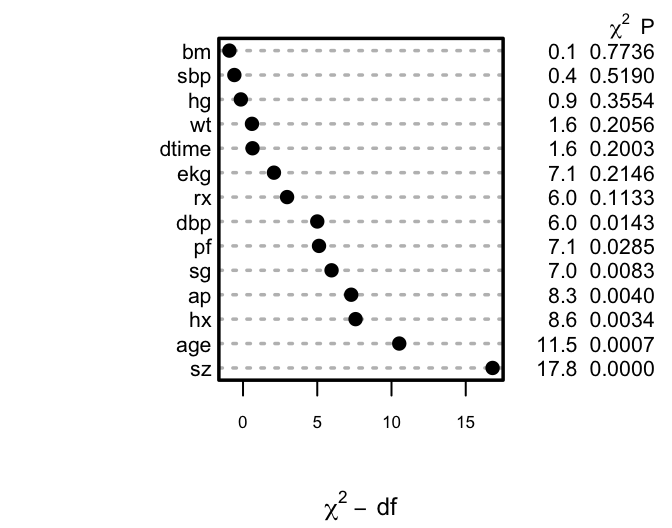Figure 11.1: Ranking of apparent importance of predictors of cause of death
Code
dd <- datadist(psub); options(datadist='dd')
ggplot(Predict(f), sepdiscrete='vertical', vnames='names',
rdata=psub,
histSpike.opts=list(frac=function(f) .1*f/max(f) ))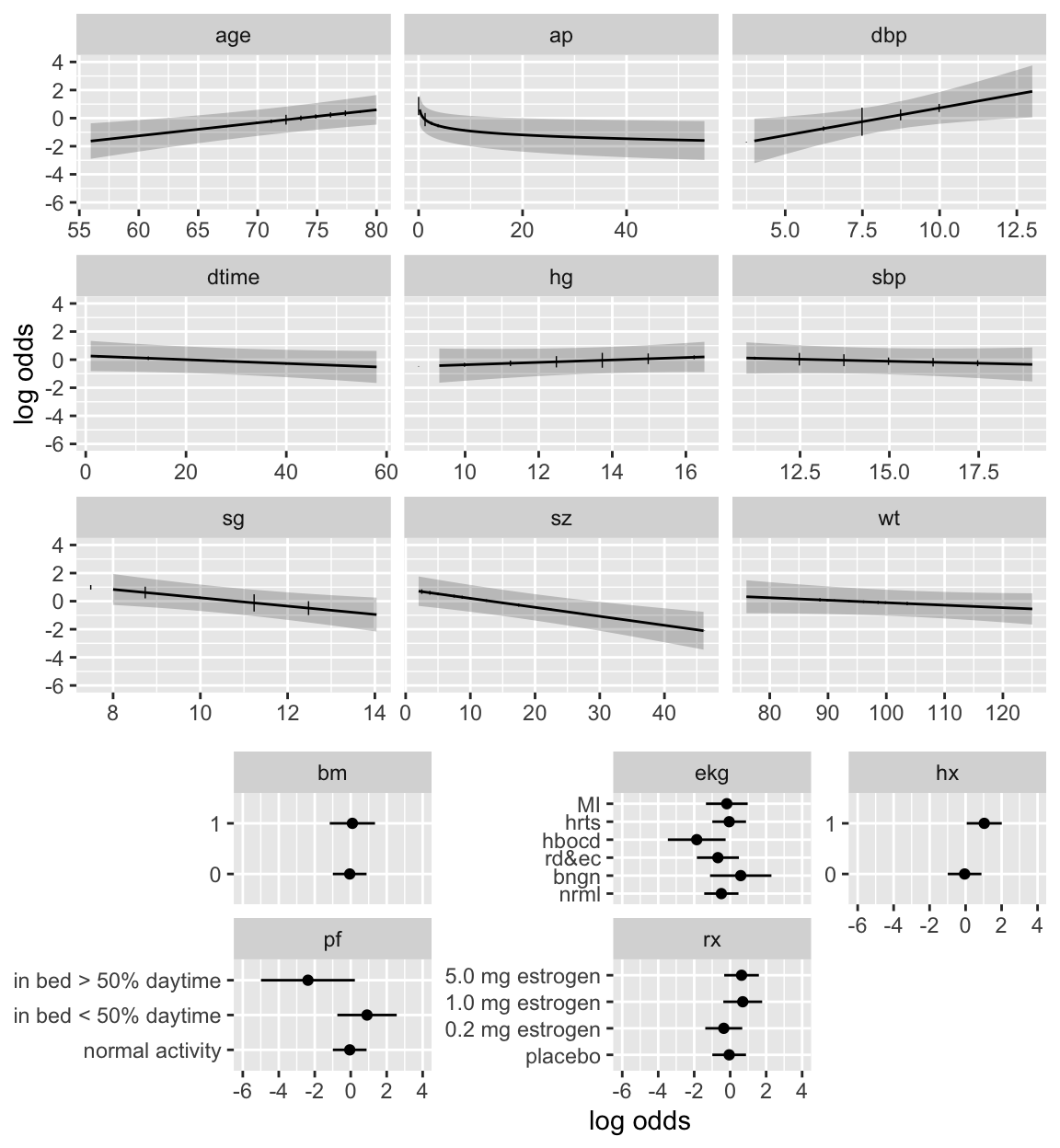Figure 11.2: Partial effects (log odds scale) in full model for cause of death, along with vertical line segments showing the raw data distribution of predictors
Code
plot(summary(f), log=TRUE)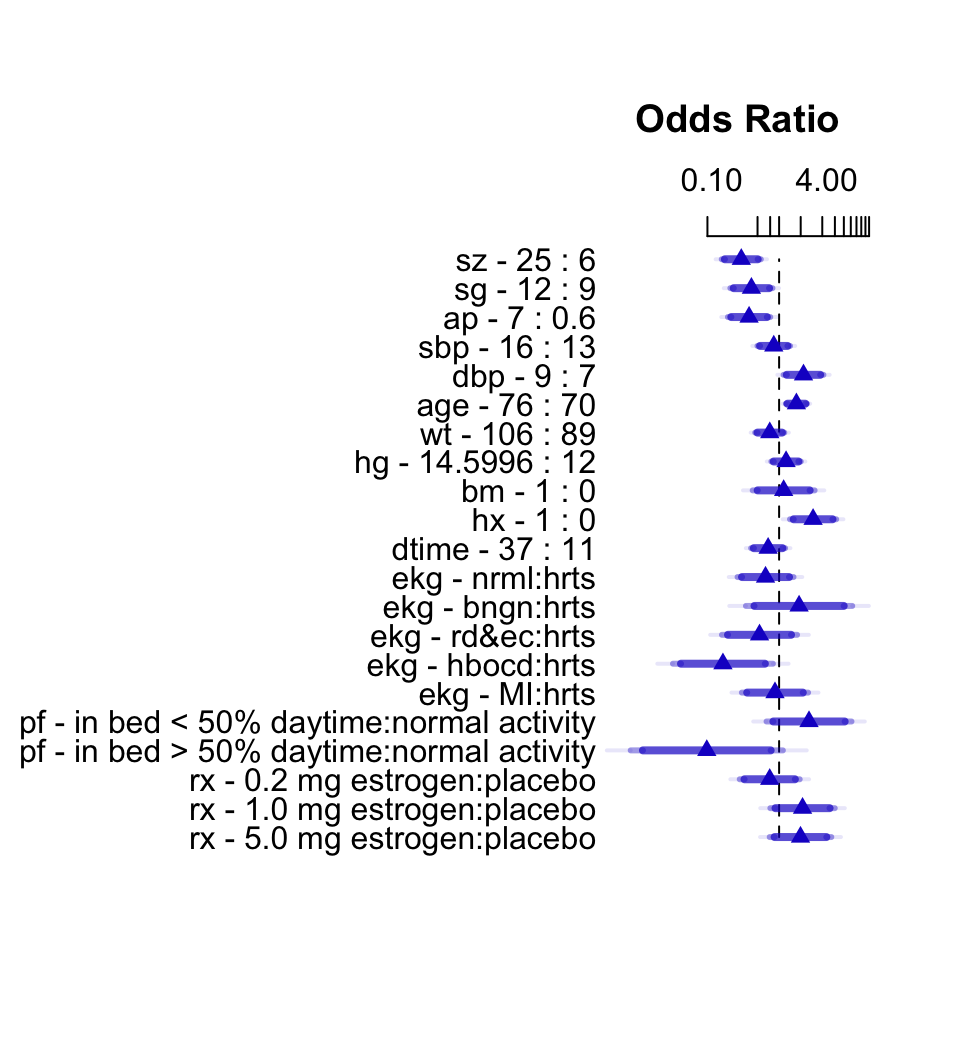Figure 11.3: Interquartile-range odds ratios for continuous predictors and simple odds ratios for categorical predictors. Numbers at left are upper quartile : lower quartile or current group : reference group. The bars represent $$0.9, 0.95, 0.99$$ confidence limits. The intervals are drawn on the log odds ratio scale and labeled on the odds ratio scale. Ranges are on the original scale.

The van Houwelingen–Le Cessie heuristic shrinkage estimate (Equation 4.1) is $$\hat{\gamma}=0.85$$, indicating that this model will validate on new data about 15% worse than on this dataset.

## 11.6 Backwards Step-Down

Now use fast backward step-down (with total residual AIC as the stopping rule) to identify the variables that explain the bulk of the cause of death. Later validation will take this screening of variables into account. The greatly reduced model results in a simple nomogram.

Code
fastbw(f)

Deleted Chi-Sq d.f. P      Residual d.f. P      AIC
ekg     6.76   5    0.2391  6.76     5   0.2391  -3.24
bm      0.09   1    0.7639  6.85     6   0.3349  -5.15
hg      0.38   1    0.5378  7.23     7   0.4053  -6.77
sbp     0.48   1    0.4881  7.71     8   0.4622  -8.29
wt      1.11   1    0.2932  8.82     9   0.4544  -9.18
dtime   1.47   1    0.2253 10.29    10   0.4158  -9.71
rx      5.65   3    0.1302 15.93    13   0.2528 -10.07
pf      4.78   2    0.0915 20.71    15   0.1462  -9.29
sg      4.28   1    0.0385 25.00    16   0.0698  -7.00
dbp     5.84   1    0.0157 30.83    17   0.0209  -3.17

Approximate Estimates after Deleting Factors

Coef    S.E. Wald Z         P
Intercept -3.74986 1.82887 -2.050 0.0403286
sz        -0.04862 0.01532 -3.174 0.0015013
ap        -0.40694 0.11117 -3.660 0.0002518
age        0.06000 0.02562  2.342 0.0191701
hx         0.86969 0.34339  2.533 0.0113198

Factors in Final Model

 sz  ap  age hx 
Code
fred <- lrm(cvd ~ sz + log(ap) + age + hx, data=psub)
latex(fred, file='')
$\Pr(\text{cvd}) = \frac{1}{1+\exp(-X\beta)}, \text{~~where} \\$ $\begin{array} \lefteqn{X\hat{\beta}=}\\ & & -5.009276 -0.05510121\:\text{sz} -0.509185\:\text{\log(ap)} +0.0788052\:\text{age} +1.070601\:\text{hx} \\ \end{array}$
Code
nom <- nomogram(fred, ap=c(.1, .5, 1, 5, 10, 50),
fun=plogis, funlabel="Probability",
fun.at=c(.01,.05,.1,.25,.5,.75,.9,.95,.99))
plot(nom, xfrac=.45)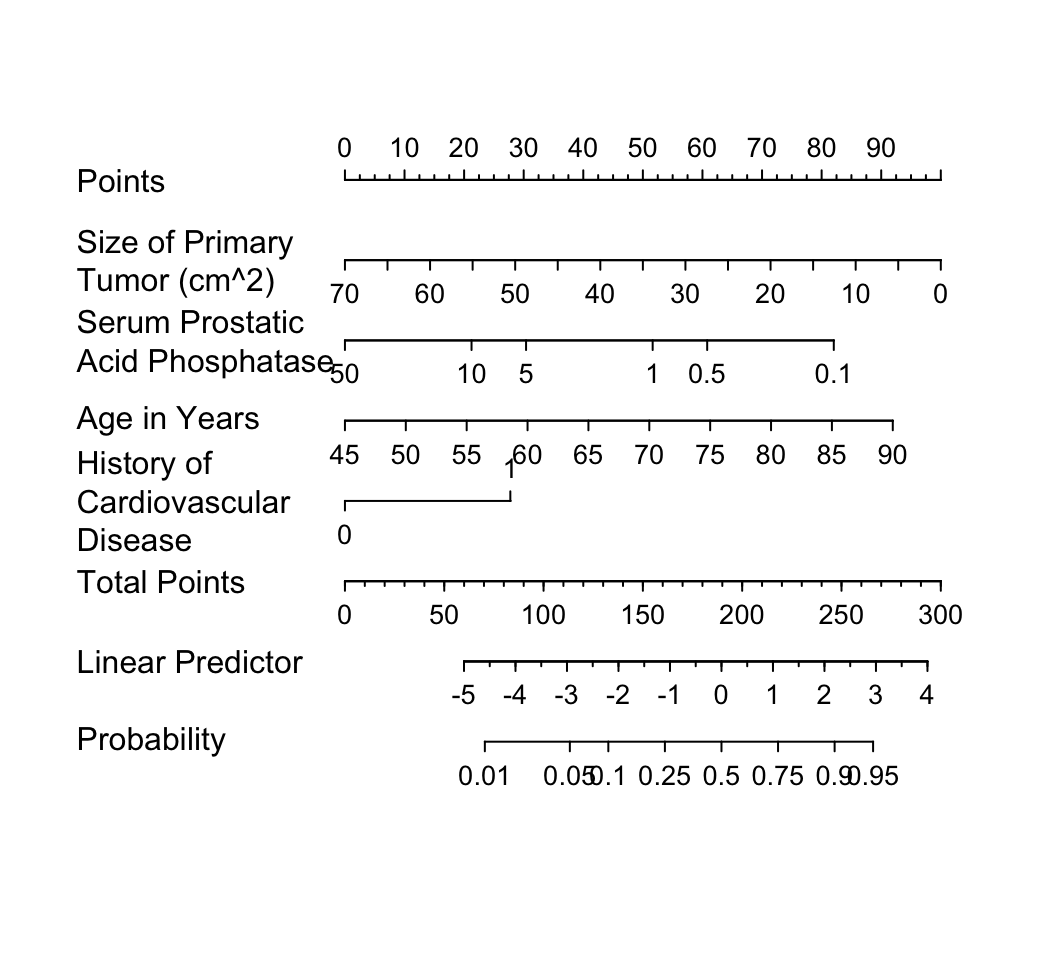Figure 11.4: Nomogram calculating $$X\hat{\beta}$$ and $$\hat{P}$$ for cvd as the cause of death, using the step-down model. For each predictor, read the points assigned on the 0–100 scale and add these points. Read the result on the Total Points scale and then read the corresponding predictions below it.

It is readily seen from this model that patients with a history of heart disease, and patients with less extensive prostate cancer are those more likely to die from cvd rather than from cancer. But beware that it is easy to over-interpret findings when using unpenalized estimation, and confidence intervals are too narrow. Let us use the bootstrap to study the uncertainty in the selection of variables and to penalize for this uncertainty when estimating predictive performance of the model. The variables selected in the first 20 bootstrap resamples are shown, making it obvious that the set of “significant” variables, i.e., the final model, is somewhat arbitrary.

Code
f <- update(f, x=TRUE, y=TRUE)
v <- validate(f, B=200, bw=TRUE)
Code
html(v, B=20, digits=3)
Index Original
Sample
Training
Sample
Test
Sample
Optimism Corrected
Index
$$n$$
$$D_{xy}$$ 0.682 0.715 0.647 0.067 0.614 200
$$R^{2}$$ 0.439 0.482 0.398 0.085 0.354 200
Intercept 0 0 0.001 -0.001 0.001 200
Slope 1 1 0.816 0.184 0.816 200
$$E_{\max}$$ 0 0 0.046 0.046 0.046 200
$$D$$ 0.395 0.448 0.352 0.097 0.298 200
$$U$$ -0.008 -0.008 0.015 -0.023 0.015 200
$$Q$$ 0.403 0.456 0.337 0.119 0.284 200
$$B$$ 0.162 0.151 0.172 -0.021 0.183 200
$$g$$ 1.932 2.213 1.777 0.436 1.496 200
$$g_{p}$$ 0.341 0.356 0.323 0.033 0.308 200
 sz sg ap sbp dbp age wt hg ekg pf bm c(“Retained in Backwards Elimination”, “ Resamples”) ⚬ ⚬ ⚬ ⚬ ⚬ ⚬ ⚬ ⚬ ⚬ ⚬ ⚬ ⚬ ⚬ ⚬ ⚬ ⚬ ⚬ ⚬ ⚬ ⚬ ⚬ ⚬ ⚬ ⚬ ⚬ ⚬ ⚬ ⚬ ⚬ ⚬ ⚬ ⚬ ⚬ ⚬ ⚬ ⚬ ⚬ ⚬ ⚬ ⚬ ⚬ ⚬ ⚬ ⚬ ⚬ ⚬ ⚬ ⚬ ⚬ ⚬ ⚬ ⚬ ⚬ ⚬ ⚬ ⚬ ⚬ ⚬ ⚬ ⚬ ⚬ ⚬ ⚬ ⚬ ⚬ ⚬ ⚬ ⚬ ⚬ ⚬ ⚬ ⚬ ⚬ ⚬ ⚬ ⚬ ⚬ ⚬ ⚬ ⚬
 1 2 3 4 5 6 7 8 9 10 11 Frequencies of Numbers of Factors Retained 6 32 52 47 33 9 11 6 2 1 1

The slope shrinkage ($$\hat{\gamma}$$) is a bit lower than was estimated above. There is drop-off in all indexes. The estimated likely future predictive discrimination of the model as measured by Somers’ $$D_{xy}$$ fell from 0.682 to 0.614. The latter estimate is the one that should be claimed when describing model performance.

A nearly unbiased estimate of future calibration of the stepwise-derived model is given below.

Code
spar(ps=9, bot=1)
cal <- calibrate(f, B=200, bw=TRUE)

Backwards Step-down - Original Model

Deleted Chi-Sq d.f. P      Residual d.f. P      AIC
ekg     6.76   5    0.2391  6.76     5   0.2391  -3.24
bm      0.09   1    0.7639  6.85     6   0.3349  -5.15
hg      0.38   1    0.5378  7.23     7   0.4053  -6.77
sbp     0.48   1    0.4881  7.71     8   0.4622  -8.29
wt      1.11   1    0.2932  8.82     9   0.4544  -9.18
dtime   1.47   1    0.2253 10.29    10   0.4158  -9.71
rx      5.65   3    0.1302 15.93    13   0.2528 -10.07
pf      4.78   2    0.0915 20.71    15   0.1462  -9.29
sg      4.28   1    0.0385 25.00    16   0.0698  -7.00
dbp     5.84   1    0.0157 30.83    17   0.0209  -3.17

Approximate Estimates after Deleting Factors

Coef    S.E. Wald Z         P
Intercept -3.74986 1.82887 -2.050 0.0403286
sz        -0.04862 0.01532 -3.174 0.0015013
ap        -0.40694 0.11117 -3.660 0.0002518
age        0.06000 0.02562  2.342 0.0191701
hx         0.86969 0.34339  2.533 0.0113198

Factors in Final Model

 sz  ap  age hx 
Code
plot(cal)

n=257   Mean absolute error=0.03   Mean squared error=0.00124
0.9 Quantile of absolute error=0.061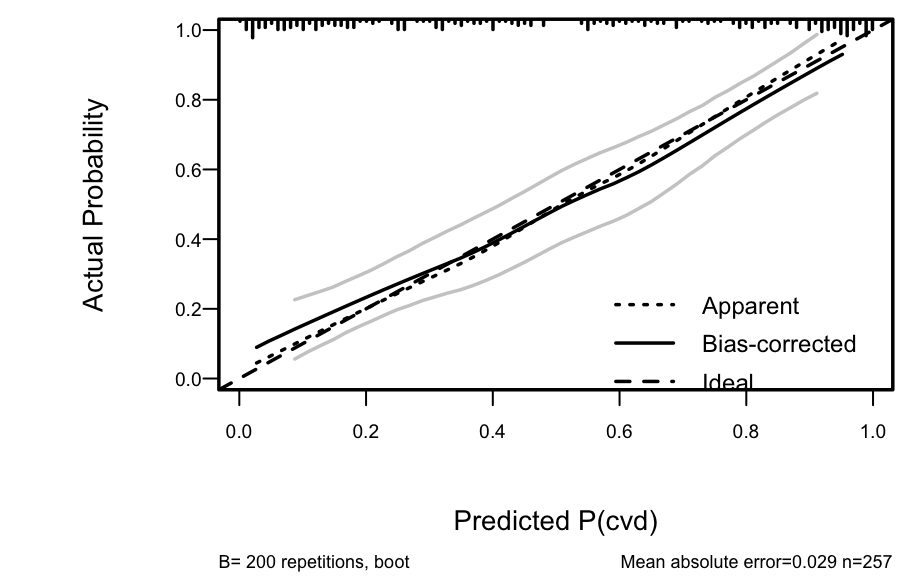Figure 11.5: Bootstrap overfitting-corrected calibration curve estimate for the backwards step-down cause of death logistic model, along with a rug plot showing the distribution of predicted risks. The smooth nonparametric calibration estimator (loess) is used.

The amount of overfitting seen in Figure 11.5 is consistent with the indexes produced by the validate function.

For comparison, consider a bootstrap validation of the full model without using variable selection.

Code
vfull <- validate(f, B=200)
html(vfull, digits=3)
Index Original
Sample
Training
Sample
Test
Sample
Optimism Corrected
Index
$$n$$
$$D_{xy}$$ 0.786 0.828 0.739 0.089 0.697 200
$$R^{2}$$ 0.573 0.635 0.503 0.132 0.441 200
Intercept 0 0 0.014 -0.014 0.014 200
Slope 1 1 0.701 0.299 0.701 200
$$E_{\max}$$ 0 0 0.081 0.081 0.081 200
$$D$$ 0.558 0.644 0.47 0.174 0.384 200
$$U$$ -0.008 -0.008 0.044 -0.052 0.044 200
$$Q$$ 0.566 0.652 0.426 0.226 0.34 200
$$B$$ 0.133 0.116 0.149 -0.033 0.166 200
$$g$$ 2.688 3.418 2.378 1.04 1.648 200
$$g_{p}$$ 0.394 0.414 0.366 0.047 0.347 200

Compared to the validation of the full model, the step-down model has less optimism, but it started with a smaller $$D_{xy}$$ due to loss of information from removing moderately important variables. The improvement in optimism was not enough to offset the effect of eliminating variables. If shrinkage were used with the full model, it would have better calibration and discrimination than the reduced model, since shrinkage does not diminish $$D_{xy}$$. Thus stepwise variable selection failed at delivering excellent predictive discrimination.

Finally, compare previous results with a bootstrap validation of a step-down model using a better significance level for a variable to stay in the model ($$\alpha=0.5$$, Steyerberg et al. (2000)) and using individual approximate Wald tests rather than tests combining all deleted variables.

Code
v5 <- validate(f, bw=TRUE, sls=0.5, type='individual', B=200)

Backwards Step-down - Original Model

Deleted Chi-Sq d.f. P      Residual d.f. P      AIC
ekg     6.76   5    0.2391  6.76     5   0.2391  -3.24
bm      0.09   1    0.7639  6.85     6   0.3349  -5.15
hg      0.38   1    0.5378  7.23     7   0.4053  -6.77
sbp     0.48   1    0.4881  7.71     8   0.4622  -8.29
wt      1.11   1    0.2932  8.82     9   0.4544  -9.18
dtime   1.47   1    0.2253 10.29    10   0.4158  -9.71
rx      5.65   3    0.1302 15.93    13   0.2528 -10.07

Approximate Estimates after Deleting Factors

Coef    S.E. Wald Z        P
Intercept               -4.86308 2.67292 -1.819 0.068852
sz                      -0.05063 0.01581 -3.202 0.001366
sg                      -0.28038 0.11014 -2.546 0.010903
ap                      -0.24838 0.12369 -2.008 0.044629
dbp                      0.28288 0.13036  2.170 0.030008
age                      0.08502 0.02690  3.161 0.001572
pf=in bed < 50% daytime  0.81151 0.66376  1.223 0.221485
pf=in bed > 50% daytime -2.19885 1.21212 -1.814 0.069670
hx                       0.87834 0.35203  2.495 0.012592

Factors in Final Model

 sz  sg  ap  dbp age pf  hx 
Code
html(v5, digits=3, B=0)
Index Original
Sample
Training
Sample
Test
Sample
Optimism Corrected
Index
$$n$$
$$D_{xy}$$ 0.739 0.802 0.718 0.083 0.656 200
$$R^{2}$$ 0.517 0.599 0.484 0.114 0.402 200
Intercept 0 0 -0.004 0.004 -0.004 200
Slope 1 1 0.748 0.252 0.748 200
$$E_{\max}$$ 0 0 0.065 0.065 0.065 200
$$D$$ 0.486 0.595 0.448 0.146 0.34 200
$$U$$ -0.008 -0.008 0.032 -0.04 0.032 200
$$Q$$ 0.494 0.602 0.416 0.187 0.307 200
$$B$$ 0.147 0.125 0.155 -0.03 0.176 200
$$g$$ 2.351 2.987 2.192 0.794 1.557 200
$$g_{p}$$ 0.372 0.401 0.36 0.041 0.331 200

The performance statistics are midway between the full model and the smaller stepwise model.

## 11.7 Model Approximation

Frequently a better approach than stepwise variable selection is to approximate the full model, using its estimates of precision, as discussed in Section 5.5. Stepwise variable selection as well as regression trees are useful for making the approximations, and the sacrifice in predictive accuracy is always apparent.

We begin by computing the “gold standard” linear predictor from the full model fit ($$R^{2} = 1.0$$), then running backwards step-down OLS regression to approximate it.

Code
spar(bty='l', ps=9)
lp <- predict(f)   # Compute linear predictor from full model
# Insert sigma=1 as otherwise sigma=0 will cause problems
a <- ols(lp ~ sz + sg + log(ap) + sbp + dbp + age + wt +
hg + ekg + pf + bm + hx + rx + dtime, sigma=1,
data=psub)
# Specify silly stopping criterion to remove all variables
s <- fastbw(a, aics=10000)
betas <- s$Coefficients # matrix, rows=iterations X <- cbind(1, f$x)    # design matrix
# Compute the series of approximations to lp
ap <- X %*% t(betas)
# For each approx. compute approximation R^2 and ratio of
# likelihood ratio chi-square for approximate model to that
# of original model
m <- ncol(ap) - 1   # all but intercept-only model
r2 <- frac <- numeric(m)
fullchisq <- f$stats['Model L.R.'] for(i in 1:m) { lpa <- ap[,i] r2[i] <- cor(lpa, lp)^2 fapprox <- lrm(cvd ~ lpa, data=psub) frac[i] <- fapprox$stats['Model L.R.'] / fullchisq
}
plot(r2, frac, type='b',
xlab=expression(paste('Approximation ', R^2)),
ylab=expression(paste('Fraction of ',
chi^2, ' Preserved')))
abline(h=.95, col=gray(.83)); abline(v=.95, col=gray(.83))
abline(a=0, b=1, col=gray(.83))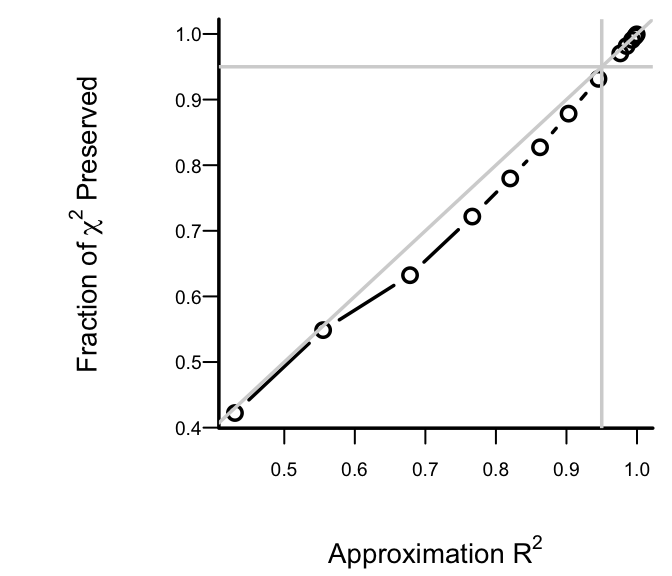Figure 11.6: Fraction of explainable variation (full model LR $$\chi^2$$) in cvd that was explained by approximate models, along with approximation accuracy ($$x$$-axis)

After 6 deletions, slightly more than 0.05 of both the LR $$\chi^2$$ and the approximation $$R^2$$ are lost. Therefore we take as our approximate model the one that removed 6 predictors. The equation for this model is below, and its nomogram is in the figure below.

Code
spar(ps=8)
fapprox <- ols(lp ~ sz + sg + log(ap) + age + ekg + pf + hx +
rx, data=psub)
fapprox\$stats['R2']   # as a check
   R2 

0.9453396

Code
latex(fapprox, file='')
$\text{E}(\text{lp}) = X\beta,~~\text{where}$ $\begin{array} \lefteqn{X\hat{\beta}=}\\ & & -2.868303 -0.06233241\:\text{sz} -0.3157901\:\text{sg} -0.3834479\:\text{\log(ap)} +0.09089393\:\text{age} \\ & & +1.396922 [\text{bngn}]+0.06275034[\text{rd\&ec}]-1.24892 [\text{hbocd}]+0.6511938 [\text{hrts}]+0.3236771 [\text{MI}] \\ & & +1.116028 [\text{in\ bed\ <\ 50\%\ daytime}]-2.436734[\text{in\ bed\ >\ 50\%\ daytime}] \\ & & +1.05316\:\text{hx} \\ & & -0.3888534[\text{0.2\ mg\ estrogen}]+0.6920495 [\text{1.0\ mg\ estrogen}] \\ & & +0.7834498 [\text{5.0\ mg\ estrogen}] \\ \end{array}$

$[c]=1~\text{if subject is in group}~c,~0~\text{otherwise}$

Code
nom <- nomogram(fapprox, ap=c(.1, .5, 1, 5, 10, 20, 30, 40),
fun=plogis, funlabel="Probability",
lp.at=(-5):4,
fun.lp.at=qlogis(c(.01,.05,.25,.5,.75,.95,.99)))
plot(nom, xfrac=.45)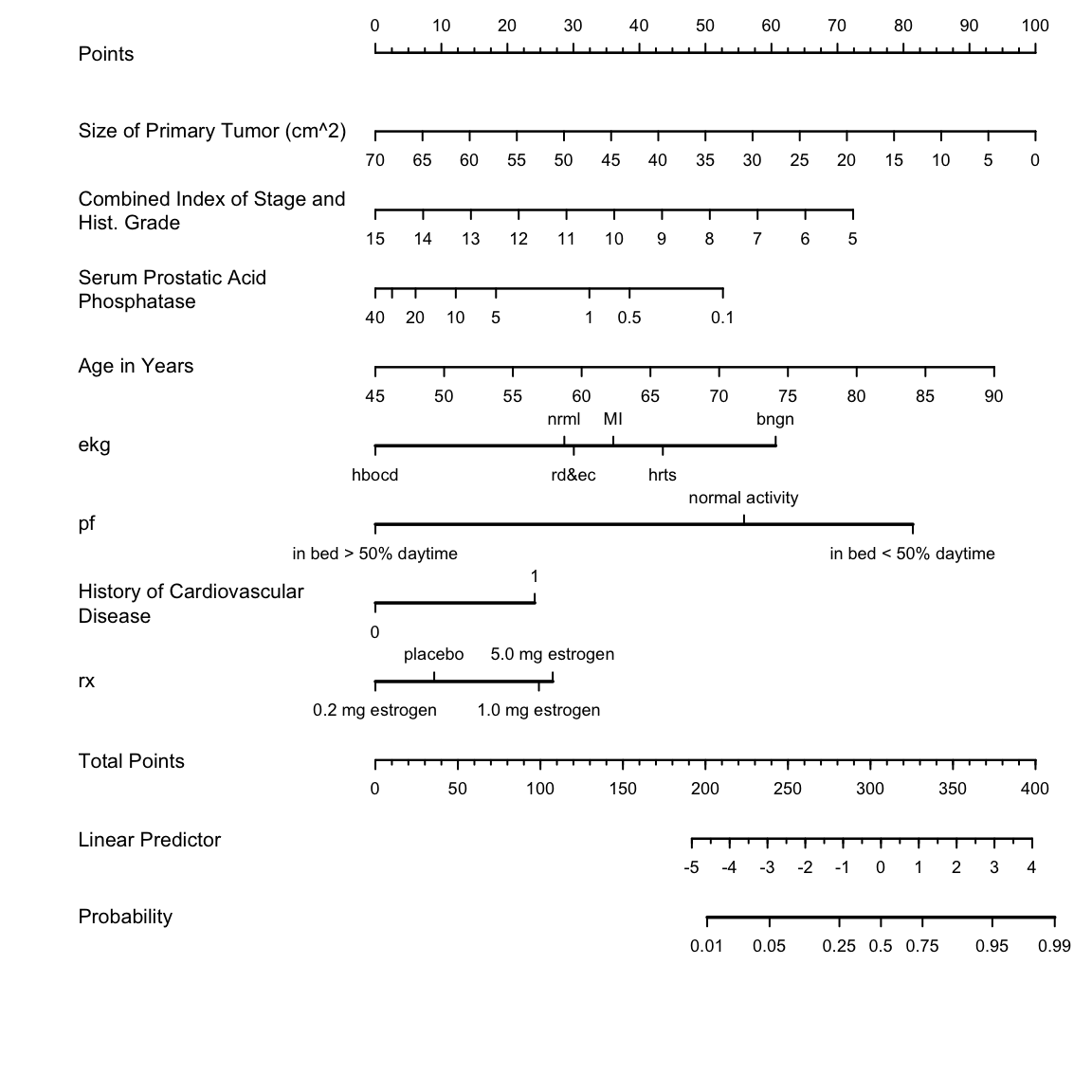Figure 11.7: Nomogram for predicting the probability of cvd based on the approximate model